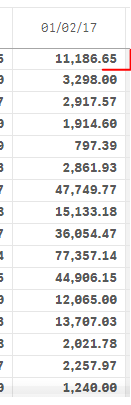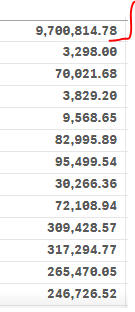# New to Qlik Sense

Discussion board where members can get started with Qlik Sense.

Announcements
Make your voice heard! Participate in the 2020 Wisdom of Crowds® Survey. BEGIN SURVEY
HighlightedNot applicable

## How can a get a sum result from averages results in a pivot table?

Hello,
I have a pivot table, with the categories and products on the left, and a column with average amounts, but when I pressed the show total button the system took me the average of the results and what I need is the SUM of the results of the average. How can I get this calculation?

Thank you.

Tags (1)
7 Replies
HighlightedMVP & Luminary

## Re: How can a get a sum result from averages results in a pivot table?

Hi,

Try aggregation function like below.

Sum(Aggr(Avg(Amount),Category,Product))

Regards,

Kaushik Solanki

Regards,
Kaushik
HighlightedMVP

## Re: How can a get a sum result from averages results in a pivot table?

May be like this

Sum(Aggr(Avg(Amount), Category, Product))

HighlightedNot applicable

## Re: How can a get a sum result from averages results in a pivot table?Where do i put the formula? in a new dimension? the first number is total (right now is calculating the average of the rest numbers in the column, i would like to have the sum in the same place. Instead of 11k, should be maybe 340k aprox.

Thank you.

HighlightedMVP

## Re: How can a get a sum result from averages results in a pivot table?

Replace your existing expression with this

Sum(Aggr(YourCurrentExpression, Category, Product))

HighlightedNot applicable

## Re: How can a get a sum result from averages results in a pivot table?

I wanted to sum just the total field.  Now is SUM everything. =SHighlightedMVP

## Re: How can a get a sum result from averages results in a pivot table?

Would you be able to share your qvf file or a sample to help you better here?Partner

## Re: How can a get a sum result from averages results in a pivot table?

Try this.

Create a variable (for example vVar).

Set the definition of the variable to =Sum(Aggr(Avg(Amount), Category, Product))